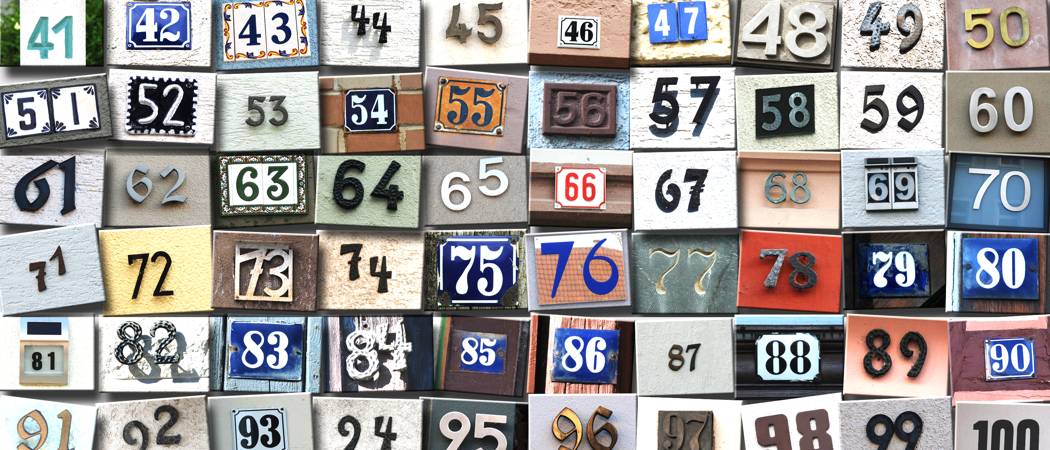# The digital sum of the products of twin primes is 8?

What connects the products of twin primes with something you learnt in primary school and the number 8?What connects the products of twin primes with something you learnt in primary school and the number 8?

Such a question might appear on a popular Radio 4 panel game called Round Britain Quiz where a panel of contestants are given a list of items that appear to have no obvious connection. So what does link these properties and why are many primary school children and their teachers unaware of digital roots or the process of casting out nines. So what is going on? Twin primes are primes that differ by two. So if $p$ and $p + 2$ are both primes then you have a twin prime. Examples are $11$ and $13$, $17$ and $19$, $29$ and $31$ Their products are $143$, $323$ and $899$. These are called semiprime as they only have two factors. If you add the digits of the products like $143$ you have $1 + 4 + 3 = 8$, $3 + 2 + 3 = 8$ and $8 + 9 + 9 = 8$. If you get a number larger than $9$, you continue with the addition. So in the case, $8 + 9 + 9 = 26$ and then you repeat this with $2$ and $6$ until you have a single-digit $2 + 6 = 8$. Is this a case of just having struck lucky?

Let us try a larger twin prime. What about $16,187$ and $16,189$. Their product is $262,051,343$ and $2 + 6 + 2 + 0 + 5 + 1 + 3 + 4 + 3 = 26$ and $2 + 6 = 8$. Again $8$. Lucky again? Why does this produce $8$ and not some other digit?First, we must have a diversion to the process of casting out nines also known as the digital root of a number. These are sometimes taught in primary school but a straw poll of a few children and a few postgraduate maths students reveals that they were unaware of it. The $9$ times table has products of $9$, $18$, $27$, $36$, $45$, $54$, $63$, $72$, $81$ and $90$ up to $10$ and you will notice that in each case the sum of the digits gives you $9$ each time. The reverse is true. If the sum of the digits of a number adds to $3$, $6$ or $9$ then the number is divisible by $3$ and if the sum is $9$ then the number is divisible by $9$. Also if not $3$, $6$ or $9$ the result will give you the remainder. Why should this be? Recall that a number in the decimal system is in the form of $a \times 10^2 + b \times 10^1 + c \times 10 ^0$ and that gives this relationship $10 ^n≡ 1 (\text{mod } 9)$. Back to our problem. So why do the digits of the products of the twin primes that we have so far tried sum to $8$? Have we just been lucky? Twin primes greater that $5$ are the form $6n \pm 1$ So if $n = 1$ we have $5$ and $7$. If $n = 2$ we have $11$ and $13$. While every twin prime is in the form of $6 n \pm 1$ the converse is not true. So when $n = 4$ we have $23$ and $25$ and while $23$ is prime, $25$ is not.

It’s still an open question if the number of twin primes is infinitely large!

Where did the relationship of $6 n \pm 1$ come from? If $p$ and $p + 2$ are both prime it follows that $p + 1$ is even. Every third number is divisible by $3$. $p – 1$ cannot be divisible by $3$, if it was it would follow that $p + 2$ would be divisible by $3$ and we know that $p + 2$ is one of our twin primes. Similarly, $p + 3$ cannot be divisible by three as that would mean $p$ was divisible by $3$ and $p$ is one of our twin primes. The only other place for $3$ is as a factor is $p + 1$ which we have found to be even and so $p + 1$ has factors of both $2$ and $3$ and so has $6$ as a factor and thus all twin primes are in the form of $6 n \pm 1$.

We still have the issue as to why the sum of the digits of the product of twin primes is $8$. Where we have a twin primes the product of $6n – 1$ and $6 n + 1$  is $36n^2 -1$ so whatever value of $n$ the product of $n$ and $36$ gives a number that has $9$ as a factor. However, in each case, we subtract $1$ and thus the sum of digits of the product of twin primes will sum to $8$.

QED.Prime numbers up to 1,000,000 in a Fermat spiral using an angle increase of 2.413, by Mario Klingemann